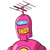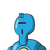# One has to run 250 metres to take one round of a ground. A girl has run 75000 centimetres. Then how many rounds has sh

One has to run 250 metres to take one round of a ground. A girl has run

75000 centimetres. Then how many rounds has she taken and how many rounds

more will she have to take in order to complete a run of 3 kilometres ?​

### 2 thoughts on “One has to run 250 metres to take one round of a ground. A girl has run <br /><br />75000 centimetres. Then how many rounds has sh”

1.One has to run 250 metres to take one round of a ground. A girl has run 75000 centimeters. Then how many rounds has she taken and how many rounds more will she have to take in order to complete a run of 3 kilometres? So, she will here to take 9 rounds more to complete 3 km.

2.Length of ground = 250m

Total distance = 75000cm = 750m

$$\frac{{750}}{{250}} = 3$$

Now, 3km = 3000m,

Out of which 750m has been covered

So, remaining distance = 3000-750 = 2250m

Again using the same formula,

$$\frac{{2250}}{{250}} = \;9$$

Hence, 9 rounds are yet to be taken